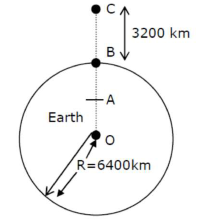# In the reported figure of earth,Question:

In the reported figure of earth, the value of acceleration due to gravity is same at point $\mathrm{A}$ and $\mathrm{C}$ but it is smaller than that of its value at point $\mathrm{B}$ (surface of the earth). The value of $\mathrm{OA}: \mathrm{AB}$ will be $x: y$. The value of $x$ isSolution:

$\frac{G M}{\left(\frac{3 R}{2}\right)^{2}}=\frac{G M r}{R^{3}}$

$O A=\frac{4 R}{9}=r$

$A B=R-\frac{4 R}{9}=\frac{5 R}{9}$

$O A: A B$

$\frac{4 R}{9}: \frac{5 R}{9} \Rightarrow 4: 5=x: y$

$(x=4)$